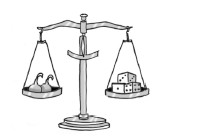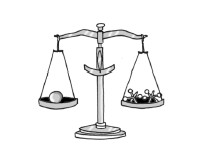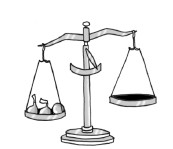### Home > AC > Chapter 6 > Lesson 6.2.2 > Problem6-53

6-53.

Examine the balanced scales in Figures 1 and 2 shown below. Figure 1 shows that two candies balance three dice. Figure 2 shows that one rubber ball balances two jacks.Figure 1Figure 2Figure 3

Determine what could be placed on the right side of the scale in Figure 3 to balance with the left side. Justify your solution in complete sentences.

$2$ candies $=3$ dice

$1$ ball $=2$ jacks

Therefore, $2$ candies $+1$ ball $=?$

$3$ dice $+2$ jacks